# NCERT Solutions for Class 9 Maths Chapter 4 Linear Equations in Two Variables| PDF Download

Here you will find Chapter 4 Linear Equations In Two Variables Class 9 Maths NCERT Solutions which are very much essential in completing homework and prepare for the exams with ease. Here you can download PDF of NCERT Solutions for Class 9 Maths Chapter 4 Linear Equations In Two Variables that will serve as beneficial tool that can be used to recall various questions any time.

These NCERT Solutions for Class 9 which are accurate and detailed are prepared by Studyrankers experts which will make learning exciting and cover the syllabus in less time. NCERT Class 9 Maths Chapter 4 will help you in rectifying all those mistakes that can happen in the exams.Page No: 68

Exercise 4.1

1. The cost of a notebook is twice the cost of a pen. Write a linear equation in two variables to represent this statement.
(Take the cost of a notebook to be x and that of a pen to be y).

Let the cost of pen be y and the cost of notebook be x.
A/q,
Cost  of a notebook = twice the pen = 2y.
2y = x
⇒ x - 2y = 0
This is a linear equation in two variables to represent this statement.

2. Express the following linear equations in the form ax + by + c = 0 and indicate the values of a, b and c in each case:
(i) 2x + 3y = 9.35               (ii) x - y/5 - 10 = 0                  (iii) -2x + 3y = 6                (iv) x = 3y
(v) 2x = -5y                        (vi) 3x + 2 = 0                        (vii) y - 2 = 0                     (viii) 5 = 2x

(i) 2x + 3y = 9.35
⇒ 2x + 3y - 9.35 = 0
On comparing this equation with ax + by + c = 0, we get
a = 2x, b = 3 and c = -9.35

(ii) x - y/5 - 10 = 0
On comparing this equation with ax + by + c = 0, we get
a = 1, b = -1/5 and c = -10

(iii) -2x + 3y = 6
⇒ -2x + 3y - 6 = 0
On comparing this equation with ax + by + c = 0, we get
a = -2, b = 3 and c = -6

(iv) x = 3y
⇒ x - 3y = 0
On comparing this equation with ax + by + c = 0, we get
a = 1, b = -3 and c = 0

(v) 2x = -5y
⇒ 2x + 5y = 0
On comparing this equation with ax + by + c = 0, we get
a = 2, b = 5 and c = 0

(vi) 3x + 2 = 0
⇒ 3x + 0y + 2 = 0
On comparing this equation with ax + by + c = 0, we get
a = 3, b = 0 and c = 2

(vii) y - 2 = 0
⇒ 0x + y - 2 = 0
On comparing this equation with ax + by + c = 0, we get
a = 0, b = 1 and c = -2

(viii) 5 = 2x
⇒ -2x + 0y + 5 = 0
On comparing this equation with ax + by + c = 0, we get
a = -2, b = 0 and c = 5

Page No: 70

Exercise 4.2

1. Which one of the following options is true, and why?
y = 3x + 5 has
(i) a unique solution,               (ii) only two solutions,             (iii) infinitely many solutions

Since the equation, y = 3x + 5 is a linear equation in two variables. It will have (iii) infinitely many solutions.

2. Write four solutions for each of the following equations:
(i) 2x + y = 7             (ii) πx + y = 9                (iii) x = 4y

(i) 2x + y = 7
⇒ y = 7 - 2x
→ Put x = 0,
y = 7 - 2 × 0 ⇒ y = 7
(0, 7) is the solution.
→ Now, put x = 1
y = 7 - 2 × 1 ⇒ y = 5
(1, 5) is the solution.
→ Now, put x = 2
y = 7 - 2 × 2 ⇒ y = 3
(2, 3) is the solution.
→ Now, put x = -1
y = 7 - 2 × -1 ⇒ y = 9
(-1, 9) is the solution.
The four solutions of the equation 2x + y = 7 are (0, 7), (1, 5), (2, 3) and (-1, 9).

(ii) πx + y = 9
⇒ y = 9 - πx
→ Put x = 0,
y = 9 - π×0 ⇒ y = 9
(0, 9) is the solution.
→ Now, put x = 1
y = 9 - π×1 ⇒ y = 9-π
(1, 9-π) is the solution.
→ Now, put x = 2
y = 9 - π×2 ⇒ y = 9-2π
(2, 9-2π) is the solution.
→ Now, put x = -1
y = 9 - π× -1 ⇒ y = 9+π
(-1, 9+π) is the solution.
The four solutions of the equation πx + y = 9 are (0, 9), (1, 9-π), (2, 9-2π) and (-1, 9+π).

(iii) x = 4y
→ Put x = 0,
0 = 4y ⇒ y = 0
(0, 0) is the solution.
→ Now, put x = 1
1 = 4y ⇒ y = 1/4
(1, 1/4) is the solution.
→ Now, put x = 4
4 = 4y ⇒ y = 1
(4, 1) is the solution.
→ Now, put x = 8
8 = 4y ⇒ y = 2
(8, 2) is the solution.
The four solutions of the equation πx + y = 9 are (0, 0), (1, 1/4), (4, 1) and (8, 2).

3. Check which of the following are solutions of the equation x - 2y = 4 and which are not:
(i) (0, 2)              (ii) (2, 0)             (iii) (4, 0)            (iv) (√2, 4√2)              (v) (1, 1)

(i) Put x = 0 and y = 2 in the equation x - 2y = 4.
0 - 2×2 = 4
⇒ -4 ≠ 4
∴ (0, 2) is not a solution of the given equation.

(ii) Put x = 2 and y = 0 in the equation x - 2y = 4.
2 - 2×0 = 4
⇒ 2 ≠ 4
∴ (2, 0) is not a solution of the given equation.

(iii) Put x = 4 and y = 0 in the equation x - 2y = 4.
4 - 2×0 = 4
⇒ 4 = 4
∴ (4, 0) is a solution of the given equation.

(iv) Put x = √2 and y = 4√2 in the equation x - 2y = 4.
√2 - 2×4√2 = 4 ⇒ √2 - 8√2 = 4 ⇒ √2(1 - 8) = 4
⇒ -7√2  ≠ 4
∴ (√2, 4√2) is not a solution of the given equation.

(v) Put x = 1 and y = 1 in the equation x - 2y = 4.
1 - 2×1 = 4
⇒ -1 ≠ 4
∴ (1, 1) is not a solution of the given equation.

4. Find the value of k, if x = 2, y = 1 is a solution of the equation 2x + 3y = k.

Given equation = 2x + 3y = k
x = 2, y = 1 is the solution of the given equation.
A/q,
Putting the value of x and y in the equation, we get
2×2 + 3×1 = k
⇒ k = 4 + 3
⇒ k = 7

Page No: 74

Exercise 4.3

1. Draw the graph of each of the following linear equations in two variables:
(i) x + y = 4                  (ii) x - y = 2               (iii) y = 3x              (iv) 3 = 2x + y

(i) x + y = 4
Put x = 0 then y = 4
Put x = 4 then y = 0
 x 0 4 y 4 0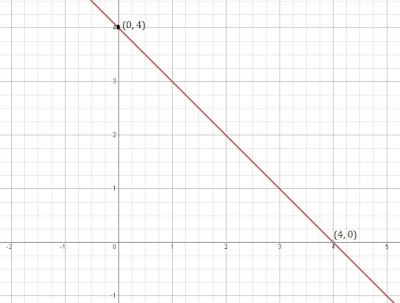(ii) x - y = 2
Put x = 0 then y = -2
Put x = 2 then y = 0
 x 0 2 y -2 0(iii) y = 3x
Put x = 0 then y = 0
Put x = 1 then y = 3
 x 0 1 y 0 3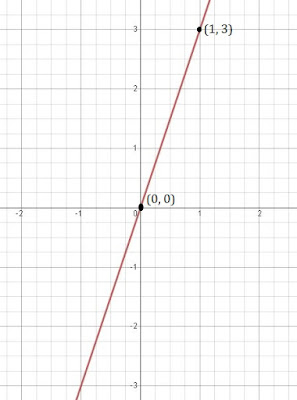(iv) 3 = 2x + y
Put x = 0 then y = 3
Put x = 1 then y = 1
 x 0 1 y 3 12. Give the equations of two lines passing through (2, 14). How many more such lines are there, and why?

Here, x = 2 and y =14.
Thus, x + y = 1
also, y = 7x ⇒ y - 7x = 0
∴ The equations of two lines passing through (2, 14) are
x + y = 1 and y - 7x = 0.
There will be infinite such lines because infinite number of lines can pass through a given point.

3. If the point (3, 4) lies on the graph of the equation 3y = ax + 7, find the value of a.

The point (3, 4) lies on the graph of the equation.
∴ Putting x = 3 and y = 4 in the equation 3y = ax + 7, we get
3×4 = a×3 + 7
⇒ 12 = 3a + 7
⇒ 3a = 12 - 7
⇒ a = 5/3

4. The taxi fare in a city is as follows: For the first kilometre, the fare is Rs 8 and for the subsequent distance it is Rs 5 per km. Taking the distance covered as x km and total fare as Rs y, write a linear equation for this information, and draw its graph.

Total fare = y
Total distance covered = x
Fair for the subsequent distance after 1st kilometre = Rs 5
Fair for 1st kilometre = Rs 8
A/q
y = 8 + 5(x-1)
⇒ y = 8 + 5x - 5
⇒ y = 5x + 3

 x 0 -3/5 y 3 0

5. From the choices given below, choose the equation whose graphs are given in Fig. 4.6 and Fig. 4.7.
For Fig. 4. 6                           For Fig. 4.7
(i) y = x                                  (i) y = x + 2
(ii) x + y = 0                           (ii) y = x – 2
(iii) y = 2x                              (iii) y = –x + 2
(iv) 2 + 3y = 7x                     (iv) x + 2y = 6

In fig. 4.6, Points are (0, 0), (-1, 1) and (1, -1).
∴ Equation (ii) x + y = 0 is correct as it satisfies all the value of the points.

In fig. 4.7, Points are (-1, 3), (0, 2) and (2, 0).
∴ Equation (iii) y = –x + 2 is correct as it satisfies all the value of the points.

Page No: 75

6. If the work done by a body on application of a constant force is directly proportional to the distance travelled by the body, express this in the form of an equation in two variables and draw the graph of the same by taking the constant force as 5 units. Also read from the graph the work done when the distance travelled by the body is
(i) 2 units               (ii) 0 unit

Let the distance traveled by the body be x and y be the work done by the force.
y ∝ x (Given)
⇒ y = 5x (To equate the proportional, we need a constant. Here, it was given 5)
A/q,
(i) When x = 2 units then y = 10 units
(ii) When x = 0 unit then y = 0 unit

 x 2 0 y 10 0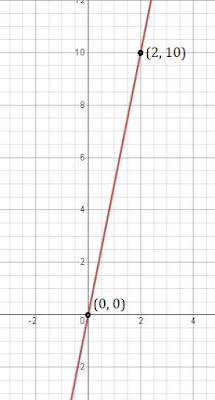7. Yamini and Fatima, two students of Class IX of a school, together contributed Rs 100 towards the Prime Minister’s Relief Fund to help the earthquake victims. Write a linear equation which satisfies this data. (You may take their contributions as Rs x and Rs y.) Draw the graph of the same.

Let the contribution amount by Yamini be x and contribution amount by Fatima be y.
A/q,
x + y = 100
When x = 0 then y = 100
When x = 50 then y = 50
When x = 100 then y = 0

 x 0 50 100 y 100 50 08. In countries like USA and Canada, temperature is measured in Fahrenheit, whereas in countries like India, it is measured in Celsius. Here is a linear equation that converts Fahrenheit to Celsius:
F = (9/5)C + 32
(i) Draw the graph of the linear equation above using Celsius for x-axis and Fahrenheit for y-axis.
(ii) If the temperature is 30°C, what is the temperature in Fahrenheit?
(iii) If the temperature is 95°F, what is the temperature in Celsius?
(iv) If the temperature is 0°C, what is the temperature in Fahrenheit and if the temperature is 0°F, what is the temperature in Celsius?
(v) Is there a temperature which is numerically the same in both Fahrenheit and Celsius? If yes, find it.

(i) F = (9/5)C + 32
When C = 0 then F = 32
also, when C = -10 then F = 14

 C 0 -10 F 32 14(ii) Putting the value of C = 30 in F = (9/5)C + 32, we get
F = (9/5)×30  + 32
⇒ F = 54 + 32
⇒ F = 86

(iii) Putting the value of F = 95 in F = (9/5)C + 32, we get
95 = (9/5)C  + 32
⇒ (9/5)C = 95 - 32
⇒ C = 63 × 5/9
⇒ C = 35

(iv) Putting the value of F = 0 in F = (9/5)C + 32, we get
0 = (9/5)C  + 32
⇒ (9/5)C = -32
⇒ C = -32 × 5/9
⇒ C = -160/9

Putting the value of C = 0 in F = (9/5)C + 32, we get
F = (9/5)× 0  + 32
⇒ F = 32

(v) Here, we have to find when F = C.
Therefore, Putting F = C in F = (9/5)C + 32, we get
F = (9/5)F + 32
⇒ F - 9/5 F = 32
⇒ -4/5 F = 32
⇒ F = -40
Therefore at -40, both Fahrenheit and Celsius numerically the same.

Page No: 77

Exercise 4.4

1. Give the geometric representations of y = 3 as an equation
(i) in one variable
(ii) in two variables

(i) in one variable, it is represented as
y = 3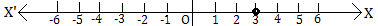(ii) in two variables, it is represented as a line parallel to X-axis.
0x + y = 3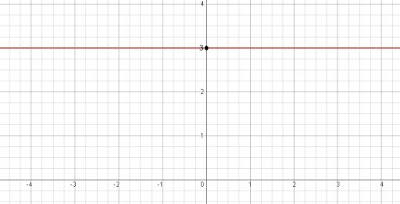2. Give the geometric representations of 2x + 9 = 0 as an equation
(i) in one variable
(ii) in two variables

(i) in one variable, it is represented as
x = -9/2(ii) in two variables, it is represented as a line parallel to Y-axis.
2x + 0y + 9 = 0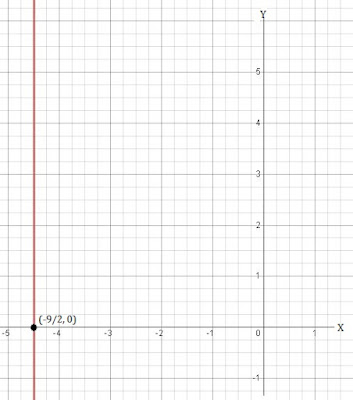## NCERT Solutions for Class 9 Maths Chapter 4 Linear Equations in Two Variables

NCERT Solutions of Chapter 4 Linear Equations in Two Variables will help in developing basic problem solving skills and scoring more marks in the exams. Any equation which can be put in the form ax + by + c = 0, where a, b and c are real numbers, and a and b are not both zero, is called a linear equation in two variables.

• Linear Equations: The solution of a linear equation is not affected when: (i) the same number is added to (or subtracted from) both the sides of the equation. (ii) you multiply or divide both the sides of the equation by the same non-zero number.

• Solution of a Linear Equation: A linear equation in two variables has infinitely many solutions. You need to find solutions of different linear equations in two variables.

• Graph of a Linear Equation in Two Variables: Every point on the graph of a linear equation in two variables is a solution of the linear equation. Moreover, every solution of the linear equation is a point on the graph of the linear equation. In this section we will look geometrical representation of the solutions of linear equations in two variables.

• Equations of Lines Parallel to the x-axis and y-axis: We need to give the geometric representations of given equations in one variable and in two variables.

There are total four exercises in the Chapter 4 Class 9 Maths textbook. You will also find exercisewise NCERT Solutions by clicking on the exercise number.

NCERT Solutions are very important to keep revising the syllabus and clearing doubts wherever occur. Through the help of the concepts, you can easily tackle the questions given the supplementary books.

### NCERT Solutions for Class 9 Maths Chapters:

 Chapter 1 Number Systems Chapter 2 Polynomials Chapter 3 Coordinate Geometry Chapter 5 Introduction to Euclid's Geometry Chapter 6 Lines and Angles Chapter 7 Triangles Chapter 8 Quadrilaterals Chapter 9 Areas of Parallelograms and Triangles Chapter 10 Circles Chapter 11 Constructions Chapter 12 Heron's Formula Chapter 13 Surface Areas And Volumes Chapter 14 Statistics Chapter 15 Probability

FAQ on Chapter 4 Linear Equations in Two Variables

#### How many exercises in Chapter 4 Linear Equations In Two Variables ?

Chapter 4 Linear Equations In Two Variables NCERT Solutions consist of four exercise which are very essential to build basic concepts and knowing how to solve a specific question type. These NCERT Solutions are updated as per the latest guidelines by CBSE.

#### What do you mean by solution of a linear equation in two variables?

A linear equation involves two variables x and y. By solution of a linear equation, we mean a pair of values, one for x and another for y which satisfy the given equation.

#### What is the equation of x-axis?

Since, the y-coordinate is zero at every point on the x-axis.
∴ The equation of x-axis is y = 0.

#### Show that x = 1, y = 3 satisfy the linear equation 3x – 4y + 9 = 0.

We have 3x – 4y + 9 = 0
Putting x = 1, y = 3, we get
L.H.S. = 3(1) – 4(3) + 9 = 3 – 12 + 9
= 12 – 12 = 0 = R.H.S.
Since, L.H.S. = R.H.S.
∴ x = 1 and y = 3 satisfy the given linear equation.